## Fun With SAS ODS Graphics, July 4th Edition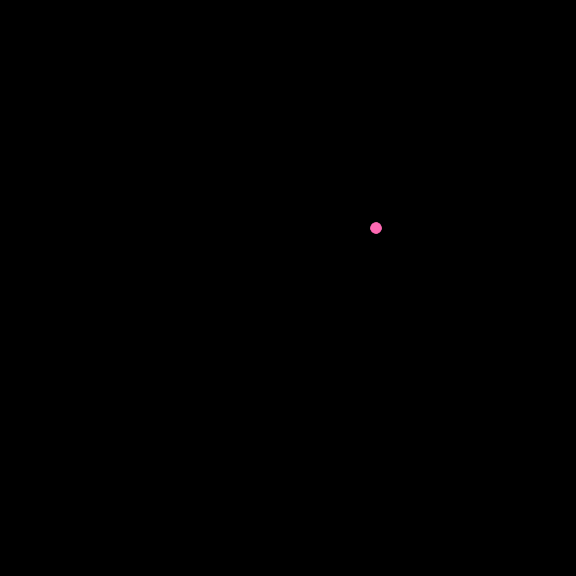OK, animated GIF GTL vector plots admittedly are not nearly as fun as the firecrackers of my youth, but at least I'll still have all my fingers at the end of the day!

Acknowledgements: If some of the code/concepts look familiar to readers of Sanjay Matange's Graphically Speaking blog posts on some real use cases for vector plots and animated plots, it's no coincidence (bugs/kludges are my own!).

SAS CODE

```* Fun with GTL vectorplots, 4th of July animated GIF "fireworks";

data fireworks;                * Generate points for fireworks;
pi=constant('pi');
do firework=1 to 7;            * Seven fireworks "explosions";
r=.2+ranuni(2)*.3;           * Random radius between .2 and .5;
if firework=7 then r=.5;     * Big finish! (radius=.5);
xo=r+ranuni(4)*(1-r*2);      * Random origin (x, y);
yo=r+ranuni(6)*(1-r*2);
do a=0 to 352.5 by 7.5;      * Line every 7.5 degrees;
x=r*cos(a*pi/180)+xo;      * Calc x and y coordinates for fully-exploded fireworks;
y=r*sin(a*pi/180)+yo;
xi1=.01*cos(a*pi/180)+xo;  * Initial unexploded x, y points (r=.01, .02);
yi1=.01*sin(a*pi/180)+yo;
xi2=.02*cos(a*pi/180)+xo;
yi2=.02*sin(a*pi/180)+yo;
output;
end;
end;

data empty;                    * Generate out-of-frame points to create "empty" frame;
x=-1; y=-1; xo=-1; yo=-1; r=1;
run;

ods _all_ close;               * Use generated points to produce animated GIF;
options papersize=('6 in', '6 in') printerpath=gif animation=start
nodate nonumber animduration=.1 animloop=YES NOANIMOVERLAY;
ods printer file='/folders/myfolders/fireworks/fourthofjuly.gif';
ods graphics / border=off width=6in height=6in imagefmt=GIF;

%macro fireworks;              * Vector plot (change pattern to "fade" fireworks;
%macro modtemplate(pattern=);
proc template;
define statgraph vectorplot;
begingraph / opaque=true border=false backgroundcolor=black;
layout overlayequated / equatetype=square opaque=true border=false backgroundcolor=black
wallcolor=black WALLDISPLAY=(FILL) commonaxisopts=(viewmin=0 viewmax=1)
xaxisopts=(display=none) yaxisopts=(display=none);
vectorplot y=y x=x xorigin=xo yorigin=yo /
endlayout;
endgraph;
end;
run;
%mend;

%do f=1 %to 7;                  * Generate frames for animated GIF;
data _null_;
call symput("linecolor",scan("hotpink orange dodgerblue yellow lime white red", &f));
run;
%modtemplate(pattern=solid); * Initial unexploded frames (2 frames);
proc sgrender data=fireworks(where=(firework=&f) drop=x y rename=(xi1=x yi1=y)) template=vectorplot;
proc sgrender data=fireworks(where=(firework=&f) drop=x y rename=(xi2=x yi2=y)) template=vectorplot;
%modtemplate(pattern=solid);
%do i=1 %to 5;               * Fully exploded frames (5 frames);
proc sgrender data=fireworks(where=(firework=&f)) template=vectorplot;
%end;                        * Fading/empty frames (3 frames);
%modtemplate(pattern=shortdashdot);
proc sgrender data=fireworks(where=(firework=&f)) template=vectorplot;
%modtemplate(pattern=dot);
proc sgrender data=fireworks(where=(firework=&f)) template=vectorplot;
proc sgrender data=empty template=vectorplot;
%end;
run;
%mend;

%fireworks;                     * Light 'em up!;
options printerpath=gif animation=stop;
ods printer close;```

LINE PATTERNS USED TO SHOW/"FADE" FIREWORKS (SOLID, SHORTDASHDOT, DOT)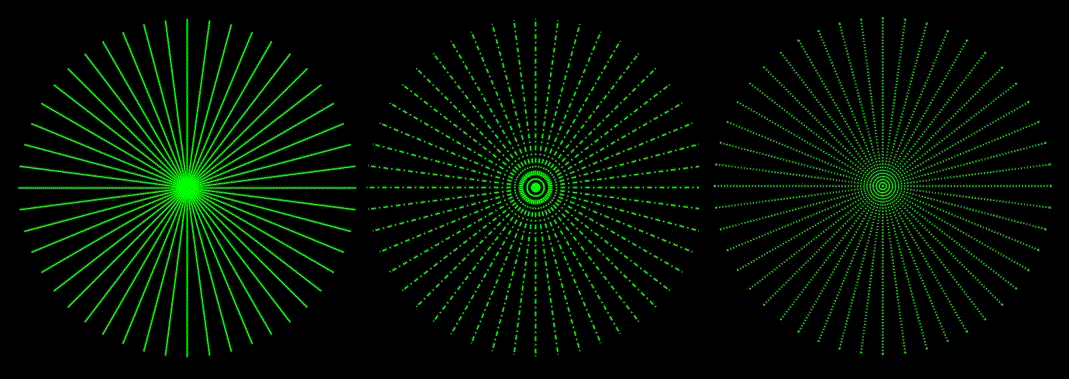13 REPLIES 13

## Re: Fun With SAS ODS Graphics, July 4th Edition

I like it! A fun application of animated plots. For another fun example, see The Game of Life animation.

One minor comment on the SAS program. Only the first random number seed is relevant in your DATA step program. Changing the seed for each call doesn't do anything.

## Re: Fun With SAS ODS Graphics, July 4th Edition

Thanks for the "random tip"!

Up until now, I think my RANUNI needs have been one-statement-per-DATA-step simple.

Like The Game of Life in SAS/IML Studio, too!Jay54
Meteorite | Level 14

## Re: Fun With SAS ODS Graphics, July 4th Edition

Awesome July 4 firework animation, Ted.  Love the way you fade out the light using line patterns.  Made my day.

## Re: Fun With SAS ODS Graphics, July 4th Edition

That's pretty cool! 🙂

## Re: Fun With SAS ODS Graphics, July 4th Edition

WOW!!!

I had ro comment out  opaque=true   'begingraph /   /*opaque=true*/  border=false backgroundcolor=black; ".

Ran nicely after that

Win 7 64 SAS 64 9.4M2

## Re: Fun With SAS ODS Graphics, July 4th Edition

Breathtaking!

May I suggest a tiny tweak? If you were to use mvar or dynamics for the vectorplot statement's pattern= and linecolor=, you can do without the macro modtemplate(). Tweaked code attached.

## Take 2: Static B/W image of a GTL vectorplot "fireworks" over downtown Chicago skylineAnd with the help of an adapted version of a Wikimedia Commons image attributed to Eduardo Manchon and a tip from an old SGF Paper of Dan Heath's, here's a simpler version of the code that generates a static B/W image of a GTL vectorplot fireworks show over the downtown Chicago skyline!

CODE

```* Chicago skyline with GTL vectorplot "fireworks";

data fireworks;                * Generate points for fireworks;
pi=constant('pi');
firework+1;                    * Assign group ID for later vector plot;
do a=0 to 352.5 by 7.5;        * Line every 7.5 degrees;
x=r*cos(a*pi/180)+xo;        * Calc x and y coordinates for fireworks;
y=r*sin(a*pi/180)+yo;
output;
end;
datalines;
.15 .13 .85
.23 .62 .775
.07 .95 .925
;
ods listing gpath='/folders/myfolders/fireworks';
ods graphics on / reset=index imagename='Chicago4thJuly' border=off
width=5in height=5in imagefmt=GIF;

data chicago;                  * Image of Chicago skyline;
retain function "Image" anchor "bottomleft" x1 0 y1 0 width 100 height 100
widthunit 'percent' heightunit 'percent' imagescale  'fit'
drawspace "layoutpercent" layer 'back'
id 'chicago' Image "/folders/myfolders/chicago.gif";

proc template;
define statgraph vectorplot;
begingraph / opaque=true border=false drawspace=layoutpercent backgroundcolor=black;
layout overlayequated / equatetype=square border=false
WALLDISPLAY=NONE commonaxisopts=(viewmin=0 viewmax=1)
xaxisopts=(display=none) yaxisopts=(display=none);
vectorplot y=y x=x xorigin=xo yorigin=yo / group=firework
annotate / id="chicago";
endlayout;
endgraph;
end;

proc sgrender data=fireworks template=vectorplot sganno=chicago;```

BACKGROUND IMAGE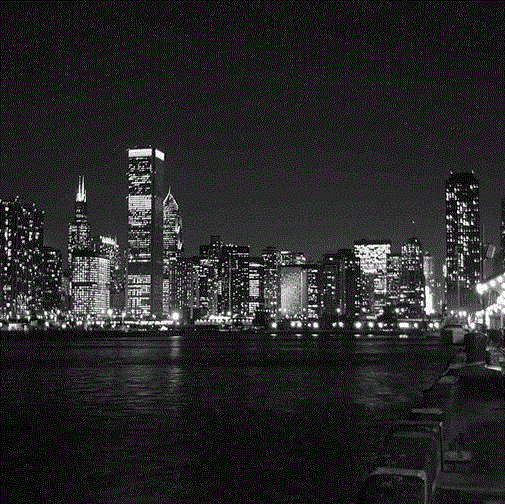## Re: Take 2: Static B/W image of a GTL vectorplot "fireworks" over downtown Chicago skyline

Having a 'blast' with your SAS graphic creativity Ted! Great work.

//Contact me to learn how Metacoda software can help keep your SAS platform secure - https://www.metacoda.com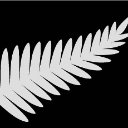ChrisNZ
Tourmaline | Level 20

## Re: Take 2: Static B/W image of a GTL vectorplot "fireworks" over downtown Chicago skyline

Oh well, someone had to cave in...

I just crudely set the sky transparent, moved the fireworks to the upper half, and shifted the image to the foreground.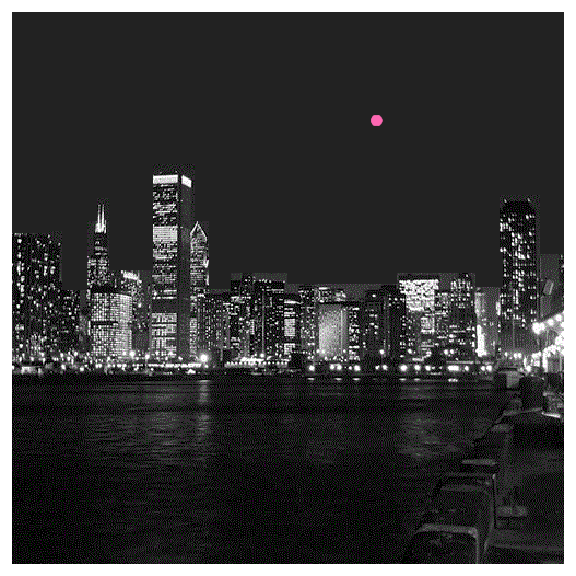Now for the reflection on the water to be added ...  🙂

File with transparent sky attached.

[Edit.

PNG file not attached as it is rejected:

The file does not have a valid extension for an attachment and has been removed. sas,txt,csv,zip,pdf,ics,sx,sxs,doc,docx,xls,xlsx,egp,sav,sas7bdat,ctm,ctk,rtf,py,spk are the valid extensions.

I added it at the bottom but it seems to lose its transparency information.

I used this website to add the transparency (color the sky red for example before uploading): https://onlinepngtools.com/create-transparent-png  ]

``````* Fun with GTL vectorplots, 4th of July animated GIF "fireworks";

data fireworks;                * Generate points for fireworks;
pi=constant('pi');
do firework=1 to 7;            * Seven fireworks "explosions";
r=.2+ranuni(2)*.3;           * Random radius between .2 and .5;
if firework=7 then r=.3;     * Big finish! (radius=.5);
xo=r+ranuni(4)*(1-r*2);      * Random origin (x, y);
yo=r+ranuni(6)*(1-r*2)/3+.4;
do a=0 to 352.5 by 7.5;      * Line every 7.5 degrees;
x=r*cos(a*pi/180)+xo;      * Calc x and y coordinates for fully-exploded fireworks;
y=r*sin(a*pi/180)+yo;
xi1=.01*cos(a*pi/180)+xo;  * Initial unexploded x, y points (r=.01, .02);
yi1=.01*sin(a*pi/180)+yo;
xi2=.02*cos(a*pi/180)+xo;
yi2=.02*sin(a*pi/180)+yo;
output;
end;
end;

data empty;                    * Generate out-of-frame points to create "empty" frame;
x=-1; y=-1; xo=-1; yo=-1; r=1;
run;

ods _all_ close;               * Use generated points to produce animated GIF;
options papersize=('6 in', '6 in') printerpath=gif animation=start
nodate nonumber animduration=.1 animloop=YES NOANIMOVERLAY;
ods printer file="%sysfunc(pathname(WORK))\fourthofjuly.gif";
ods graphics / border=off width=6in height=6in imagefmt=GIF;

%macro fireworks;              * Vector plot (change pattern to "fade" fireworks;
%macro modtemplate(pattern=);
proc template;
define statgraph vectorplot;
begingraph ;*/ opaque=true border=false backgroundcolor=black;
layout overlayequated / equatetype=square opaque=false border=false backgroundcolor=cx222222
wallcolor=cx222222 WALLDISPLAY=(fill) commonaxisopts=(viewmin=0 viewmax=1)
xaxisopts=(display=none) yaxisopts=(display=none);
vectorplot y=y x=x xorigin=xo yorigin=yo /
annotate / id="chicago";  endlayout;
endgraph;
end;
run;
%mend;

%do f=1 %to 7;                  * Generate frames for animated GIF;
data _null_;
call symput("linecolor",scan("hotpink orange dodgerblue yellow lime white red", &f));
run;
%modtemplate(pattern=solid); * Initial unexploded frames (2 frames);
proc sgrender data=fireworks(where=(firework=&f) drop=x y rename=(xi1=x yi1=y)) template=vectorplot sganno=chicago;;
proc sgrender data=fireworks(where=(firework=&f) drop=x y rename=(xi2=x yi2=y)) template=vectorplot sganno=chicago;;
%modtemplate(pattern=solid);
%do i=1 %to 5;               * Fully exploded frames (5 frames);
proc sgrender data=fireworks(where=(firework=&f)) template=vectorplot sganno=chicago;
%end;                        * Fading/empty frames (3 frames);
%modtemplate(pattern=shortdashdot);
proc sgrender data=fireworks(where=(firework=&f)) template=vectorplot sganno=chicago;
%modtemplate(pattern=dot);
proc sgrender data=fireworks(where=(firework=&f)) template=vectorplot sganno=chicago;
proc sgrender data=empty template=vectorplot sganno=chicago;
%end;
run;
%mend;

data chicago;                  * Image of Chicago skyline;
retain function "Image" anchor "bottomleft" x1 0 y1 0 width 100 height 100
widthunit 'percent' heightunit 'percent' imagescale  'fit'
drawspace "layoutpercent" layer 'fore'
id 'chicago' Image "%sysfunc(pathname(WORK))\ch.png";
run;

%fireworks;                     * Light 'em up!;
options printerpath=gif animation=stop;
ods printer close;``````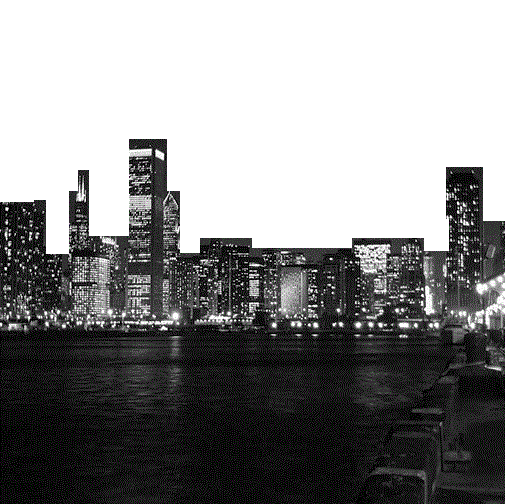## Re: Fun With SAS ODS Graphics, July 4th Edition

Thanks for sharing the fantastic animation.  I create the gif file by using your SAS code. But I don't know how to insert into Power Point slide to display. Could you enlighten me on it?

EthanChrisNZ
Tourmaline | Level 20

## Re: Fun With SAS ODS Graphics, July 4th Edition

> But I don't know how to insert into Power Point slide to display.

Just insert as a normal image.

The animation only shows in full screen mode.ChrisNZ
Tourmaline | Level 20

## Re: Fun With SAS ODS Graphics, July 4th Edition

With reflections!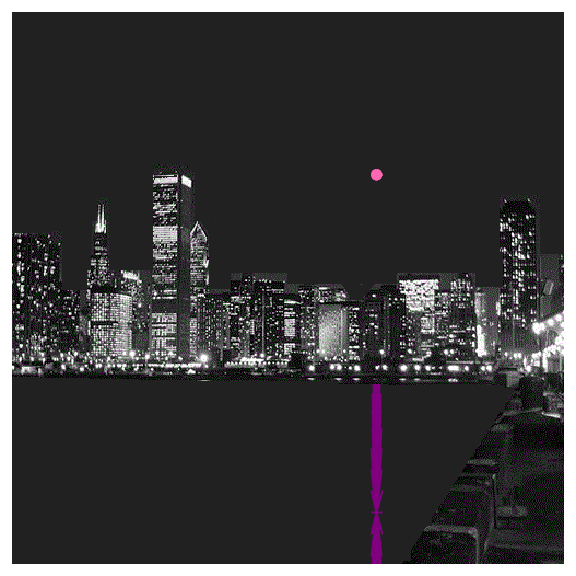``````* Fun with GTL vectorplots, 4th of July animated GIF "fireworks";

data f;                * Generate points for fireworks;
pi=constant('pi');
do firework=1 to 7;            * Seven fireworks "explosions";
r=.2+ranuni(2)*.3;           * Random radius between .2 and .5;
if firework=7 then r=.5;     * Big finish! (radius=.5);
xo=r+ranuni(4)*(1-r*2);      * Random origin (x, y);
yo=r+ranuni(6)*(1-r*2)/3+.3;
do a=0 to 352.5 by 7.5;      * Line every 7.5 degrees;
x=r*cos(a*pi/180)+xo;      * Calc x and y coordinates for fully-exploded fireworks;
y=r*sin(a*pi/180)+yo;
xi1=.01*cos(a*pi/180)+xo;  * Initial unexploded x, y points (r=.01, .02);
yi1=.01*sin(a*pi/180)+yo;
xi2=.02*cos(a*pi/180)+xo;
yi2=.02*sin(a*pi/180)+yo;

*reflection;
waterline=.4;
h=yo-waterline;
yyo=yo-h*2;  yy=y-h*2;

*clip low Y;
newy=max(y,waterline);
ratio=coalesce(divide(y-yo,newy-yo),1); x=xo+(x-xo)/ratio;  y=newy;

output;
end;
end;

data empty;                    * Generate out-of-frame points to create "empty" frame;
retain x y xo yo yy yyo -1 ;
run;

ods _all_ close;               * Use generated points to produce animated GIF;
options papersize=('6 in', '6 in') printerpath=gif animation=start
nodate nonumber animduration=.1 animloop=YES NOANIMOVERLAY;
ods printer file="%sysfunc(pathname(WORK))\j4.gif";
ods graphics / border=off width=6in height=6in imagefmt=GIF;

%macro fireworks;              * Vector plot (change pattern to "fade" fireworks;
%macro modtemplate(pattern=);
proc template;
define statgraph vectorplot;
begingraph ;
layout overlayequated / equatetype=square walldisplay=(fill) wallcolor=cx222222
commonaxisopts=(viewmin=0 viewmax=1) xaxisopts=(display=none) yaxisopts=(display=none);
vectorplot y=y x=x xorigin=xo yorigin=yo /
annotate / id="chicago";
vectorplot y=yy x=x xorigin=xo yorigin=yyo /
endlayout;
endgraph;
end;
run;
%mend;

%do f=1 %to 7;                  * Generate frames for animated GIF;
%let linecolor=%scan(hotpink orange dodgerblue yellow lime white red, &f);
%let linecolor2=%scan(verydarkpurple verydarkorange verydarkblue verydarkyellow verydarkgreen verydarkgrey verydarkred, &f);
%modtemplate(pattern=solid); * Initial unexploded frames (2 frames);
proc sgrender data=f(where=(firework=&f.) drop=x y rename=(xi1=x yi1=y)) template=vectorplot sganno=chicago;
proc sgrender data=f(where=(firework=&f.) drop=x y rename=(xi2=x yi2=y)) template=vectorplot sganno=chicago;
%modtemplate(pattern=solid);
%do i=1 %to 5;               * Fully exploded frames (5 frames);
proc sgrender data=f(where=(firework=&f.)) template=vectorplot sganno=chicago;
%end;                        * Fading/empty frames (3 frames);
%modtemplate(pattern=shortdashdot);
proc sgrender data=f(where=(firework=&f.)) template=vectorplot sganno=chicago;
%modtemplate(pattern=dot);
proc sgrender data=f(where=(firework=&f.)) template=vectorplot sganno=chicago;
proc sgrender data=empty template=vectorplot sganno=chicago;
%end;
run;
%mend;

data chicago ;                  * Image of Chicago skyline;
retain function "Image" anchor "bottomleft" x1 0 y1 0 width 100 height 100
widthunit 'percent' heightunit 'percent' imagescale  'fit'
drawspace "layoutpercent" layer 'fore'
id 'chicago' Image "%sysfunc(pathname(WORK))\ch2.png";
run;

%fireworks;                     * Light 'em up!;
options printerpath=gif animation=stop;
ods printer close;``````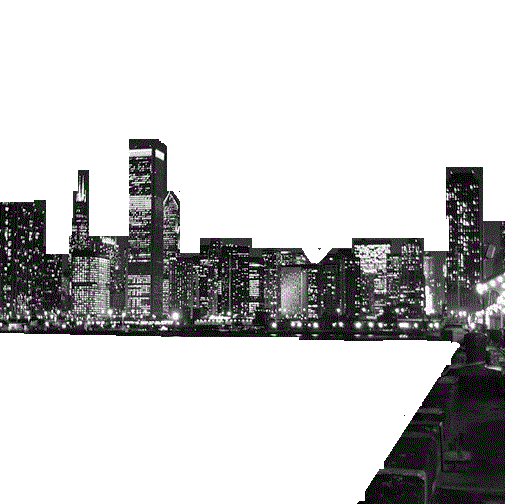the image with a transparent color

## Re: Fun With SAS ODS Graphics, July 4th Edition

Thanks to the community and especially @tc for this -- we adapted the fireworks to celebrate the 10,000 followers on the sassoftware Instagram handle.  Props!

Discussion stats
• 13 replies
• 3719 views
• 19 likes
• 10 in conversation